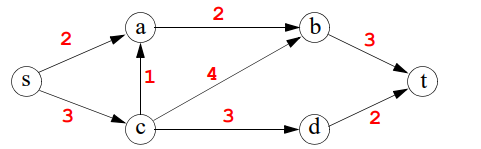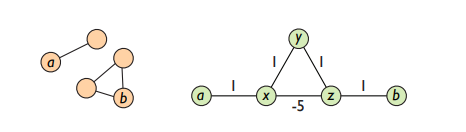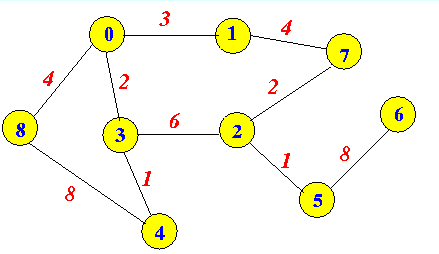# Exploring the different types of weights in a connected graph

Finding the shortest path in a network is not always the fewest nodes that need to be travelled through to a given destination. This is only applicable to an unweighted graph. For a weighted graph, the shortest path between two nodes is the least total of weight that exists between these nodes. The weights attributed to the edges of these graphs are numerical in nature and positive figures. Negative weights will prevent the determination of a minimal path. A weighted graph is viewed as a labeled graph due to the numbers assigned to each edge.

In mathematics, a weight is used to express a set of multiplicative constants allocated in front of terms in an edge of a tree. For example, the weight of a graph at a point [n] is the maximum number of edges in a given branch [n]. The weights of a graph are computed and analysed in light of the problem investigated. Weighted graphs are believed to be present in different graph scenarios such as shortest path, centrality and travelling salesman problems.

The weight of a graph assigns value to either the node or the relationship between nodes. These values determine the shortest path or most central node in a given network. The nature or type of weight is more relevant in the type of network. For example, a weight type flow may be more applicable to traffic in a computer network, fluids in a water pipe, currents in an electrical circuit or demand movements. We will look into the flow network as it pertains to weight in the subsequent section.

Different Types of graph weights

Weighted graphs are labeled graphs with positive numbers as labels. These weights can be more relevant to a certain type of network than others.

• Flow Weights: Graphs containing this type of weight are usually referred to as a flow network and are directed in nature. The amount of a flow that travels through an edge should never exceed the capacity of the respective node. It is expected that the amount of flow into a node equals the amount of flow out of it. The only occasion when this is not expected to hold is when the node is a source. A source node usually demonstrates an outgoing flow which is important in understanding the roles and importance of nodes in this type of labelled graph. On the other hand, a node only designed to receive flow is referred to as a sink. Earlier, I’ve mentioned different scenarios where a flow network can be most relevant. A good example will be the tube or train networks. Each station can be viewed as a node with respective capacity for trains or passengers to travel through. With this example, no station is a source or sink, as they all experience incoming and outgoing flow of trains and passengers. A mathematical representation of this weight can be initially represented with a graph  G = (V, E), with V being a set of nodes and E the set of edges that are connected to the nodes E. The capacity function looks at the subset of two nodes in a linear map that results to c: V × V → ℝ. These nodes may be distinct but related in the network and are considered to be connected by the same edge or members of a set of edges. In mathematical terms,  if (v, u) ∈ E then (u, v) is also a member of E. This indicates that the two nodes are both connected by the same vertex E. Finally, the capacity function of the two nodes is expressed as c(v, u) = 0. The capacity of both nodes is expected to equal zero as the amount of flow out of each node equals the inflow. None of the above nodes is viewed as a source or sink. A flow network with a source and sink is represented as (G, c, s, t). Where G is the graph, c  the capacity,  s the source and t the sink or target.
• Capacity Weight: Flow and capacity tend to mostly work together in a weighted directed graph model. The capacity weight is quite applicable to a traffic network. A good example will be a transportation network where nodes are interchanges or junctions and the edges are the respective highway segments. The edge weights are capacities related to the maximum flow of traffic a vertex or interchange segment can carry. In other words, the capacity weights of these edges determine how many cars can travel through.

The capacity weighted graph below indicates the flow from each node. The node s is the source and t is the sink. The largest capacity is edge [c,b] while the least is vertex [c,a].• Frequency weights: Traditionally frequency weighting has been applied to communications engineering for sound level measurement. More specifically in the measurement of the sound level (low or high) to the human ears. Its association to spectral analysis has led its conversion of time series into a range of frequencies. In recent times, frequency as weights is now utilised in a social network or human interaction settings. In this scenario, weights are applied between two friends, contacts or persons in line with interpersonal communication. When two individuals or nodes in a graph sense, communicate regularly, the frequency weight of the edge that connects them will be stronger and viewed as the minimal path.
• Distance weights:  In normal terms, the distance between two nodes on a graph is the number of edges in the shortest path. Distance as a form of weight in a weighted graph looks at the shortest walk. For a given graph G(V,E), w(a,b) represents the weight of edge e=(a,b). Assuming G(V,E,w) is an undirected weighted graph, it will be expected that w(a,b) = w(b,a) for (a,b) to be a ∈ (set member of) E. This type of weight can be applied to places or a transport network where the distance between two nodes (a,b) can be depicted in the weight function of w. For a vertex e = (a,b) ∈ E, the given weight of the edge determines the distance from a to b. There is a possibility that the time it requires to travel from a to b may not necessarily be the same from b to a. Resulting in a representation of w(a, b) ≠ w(b, a). The below are two graphs where we can estimate the distance between nodes a to b. The first network is a disconnected graph which indicates d(a, b) = ∞ (infinity). There is no weight distribution between both nodes due to a disconnected edge. On the other hand, the second graph is a connected one but the edge distribution of (x, y, z, x) highlights there is no minimum weight path from a to b due to the presence of a negative weight between x and z.Distance as the weight can work closely with time. This will be touched in the next section.

• Time weights: The time required to travel from two nodes in a weighted graph can determine the shortest path. As earlier stated, for a vertex e = (a,b) ∈ E, the time required to travel from a to b, may not necessarily be the same as b to a. They can both have the same edge distance but have varying time weights. For example in transport networks, one travel path may require more time due to bad road traffic, an accident or too many truck drivers due to a factory nearby. These are some of the factors that can determine the time weight between two nodes in a connected graph.
• Resistance weights: In a connected graph the resistance matrix is represented by R, and it is conveyed as R=(rij). In this instance, rij is the resistance distance between the nodes i and j of G.  For a friendship or social network a resistance in weight form could be a profile that consistently ignores replying to a message from a given user. Or, in the case of LinkedIn, ignoring to accept a connection request. The least resistance between two nodes could be the shortest path in a connected graph.
• Cost weights: This looks at the amount of effort required to travel from one place to another in a connected network. It could be financial, material or human capital in nature. One could compare the cost of travelling from London to New York from a cost perspective. The different airlines can be different edges while the nodes are the source, transit and destination.

Example from the graph below, London can be represented by a node (0) and New York as (4). There are two airlines as edges (0,8,4) and (0,3,4). Nodes (8) and (3) are the transit airports en route to Newyork. This example reveals the most cost-effective airline is (0,3,4) with a combined cost of 3.The above are seven examples of how weights could be represented in a connected weighted network.